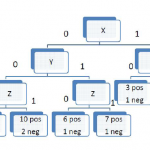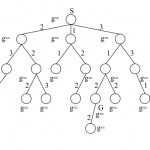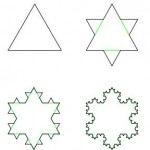# Labs

## Lab 12: :)

Part I: Project Work on your project! Part II: Project Work on your project some more! By now, you should be able to thoroughly explain to me what your topic is about and how it works if I were to sit down and talk with you for half an hour. Part III: Project Work on it some [...]

## Lab 11: Bayesian Networks

Part I: Probability Linearity of Expectation can be summarized as E(aX+bY) = aE(X) + bE(Y). Prove this using the facts we have learned so far. Prove the Tower Rule, E(E(X|Y)) = E(X) Variance and Covariance Show that E(XY)=E(X)E(Y) is not a validity. When does E(XY)=E(X)E(Y)? The difference, E(XY)-E(X)E(Y) is called the covariance. Why is this [...]

## Lab 10: Association, Clustering, and Probability

Part 0: RAWR At this point, you should most definitely NOT be working on Pathfinder anymore, unless you have completed everything else. You should make sure that, at the bare minimum, the following important basics are complete, in this order, before you move on to anything else: (1) Lab 6, Part I. (2) Lab 6, [...]## Lab 9: Learning Theory and non-parametric learning

Part 0: Learning Theory You are making the classifier for faces, and run your first test, and discover that your test error is 0.42, and training error is 0.35. What does this mean in terms of what the error actually is? What tests can you run to see what the cause of the error is? [...]

## Lab 8: Decision Trees and Learning Theory

Part I: Learning In our general algorithm for handling decision trees, we can’t handle the case when the attribute value is missing. In real life, this happens all the time. This could happen because the datum was not collected, the datum had an error and was removed, or many other real-world reasons. Given the general [...]

## Lab 7: Intro to Machine Learning

Part 0: Machine Learning Formulation For each of the following learning problems, answer these questions. Would you use regression or classification? What are good features? What could an evaluation function be? What would the optimal (maximum or minimum) correspond to? Are there local minima or maxima to be aware of? Predicting whether a drink was [...]

## Lab 6: Big-O and Project Scoping

Part I: Project Topic! If any of the following are true, please do not do anything else before resolving it. You do not have a topic. You have a topic, but have not posted the half-page to a page of preliminary research to the blog. You have posted to the blog, but Lekan has not [...]## Lab 5: Heuristic Search

Part I: Proofs Prove the following: (Direct Proof Warmup) Prove that every odd integer is the difference of two square numbers. (Proof by Contradiction Warmup) Prove that there is no largest rational number. Prove or disprove, that, if is odd, then n is odd. In logic, the contrapositive of a statement is defined as . [...]## Lab 4: Recursion and Pathfinder Search

Part 0: Data Structure (Optional) This part is completely optional, but if you are having trouble with data structures, these three short exercises may help you understand them better. As a reminder, to create random numbers, declare a Random r = new Random() as an instance variable, then call r.nextInt(), r.nextDouble(), or r.nextBoolean(). Create a [...]

## Lab 3: Math, Logic, and More Pathfinder

Part I: Fun with Math Functions and their properties For the following functions, state whether or not an inverse exists. If so, give it. If not, state why not. Show that if is a bijection, then . (Challenge) Prove that if f is a bijection, then is a bijection. Series Find a closed-form expression for [...]